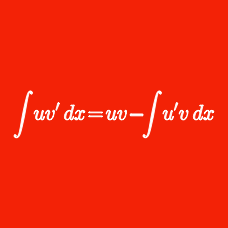Calculus

# Integration Techniques: Level 3 Challenges

Suppose that $f$ is a smooth function (meaning that all orders of derivatives exist and are continuous) such that $f(0)=1$, $f(2)=3$ and $f' (2) = 5$, then evaluate

$\large \int_{0}^{1} x f'' (2x)\, dx .$

The value of the integral $\int_0^{\pi/2}\dfrac{\text dx}{2+\cos x}$ can be expressed in the form $\dfrac{\pi^A\sqrt{B}}{C}$ where $A$, $B$, $C$ are positive integers and $B$ is not divisible by the square of any prime. Find the value of $A+B+C.$

$\int_3^6 \left ( \sqrt{x + \sqrt{12x-36}} + \sqrt{x - \sqrt{12x-36}} \ \right ) \ dx$

If the definite integral above can be expressed as $a \sqrt b$ where $a,b$ are positive integers with $b$ square free, then what is the value of $ab$?

Evaluate the integral $\displaystyle \int_{0}^{\infty} \frac{\ln x}{x^2+2x+4} \, dx.$

$\large \displaystyle\int_{\frac{\pi}{6}}^{\frac{\pi}{3}} \frac{\cot(x)\sec^2(x)}{\cot(x) + 1}\, dx$